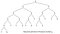# Solving Fibonacci: Recursion

It’s once again time to return to everybody’s favorite algorithm: the fibonacci sequence.

Now, before we begin I know what you are probably thinking: but Hope, we already solved fibonacci! And yes, you would be right, however this time we are going to be taking a completely different approach to our fibonacci solution, one that very well might help you ace a technical interview.

Today, our new technique will be one you might have heard of, you might be really familiar with or be completely frightened by, but regardless it’s one to know and understand well when it comes to preparing for technical interviews: recursion.

Quick Fibonacci Recap

If you’ve never heard of the fibonacci sequence before or never attempted to write a solution to the fibonacci algorithm, I would check out my previous blog: Solving Fibonacci: Iteration. Personally, I find the iterative solution to this problem to be significantly more intuitive and easier to understand, so if you’re new, I think it’s a better place to start.

However, regardless if this is your first experience with fibonacci or you thirtieth, I think it will be helpful to clarify what the fibonacci sequence is and the goal of our algorithm.

Basically, the goal of the fibonacci algorithm is to print out the nth entry in the fibonacci series. The fibonacci series/sequence is a series of numbers in which each number is the sum of the two preceding numbers.

Last time, we used a relatively straightforward iterative solution to solve this problem, but today we are going to take a look at the other common way to solve this algorithm: recursion.

What is recursion?

Recursion might seem elusive. It might seem frightening. It might seem impossibly complicated. And it is. All of those things. But, unfortunately it also a helpful way to solve algorithms and a technique you should definitely be able to implement during a technical interview, so I will try to break it down simply to help you understand it a bit better.

Basically, recursion is a method where the solution depends on solutions to small instances of the same problem. If you are still feeling a bit lost by the explanation, don’t worry. The recursive solution to fibonacci is a great example of recursion in general and as we work through the problem I am sure you will get a better understanding of this concept!

The solution

`function fib(n) {      if (n < 2) {      return n   }   return fib(n-1) + fib(n-2) }`

So, this is the solution and while it make look simple (only four lines of code!), you are probably very confused as to what’s really happening. Don’t worry. Confusion is par for the course when dealing with recursion. Also, we will go over this solution in depth and really break down how it works.

The solution: What is actually happening

The first thing to notice about our solution is that the only time we will actually return a number, is when n is less than two (so n is either 0 or 1). Also remember that when we call fibonacci of 0, we get back 0 and when we call fibonacci of 1, we get back 1 (see the chart of fibonacci numbers, above).

With that in mind, let’s say that n is 5. Well, the first thing our algorithm will check is to see if n is less than 2, which since it’s 5, obviously it’s not. Then, our algorithm will make two more calls to itself (recursion!) with fib(5–1) and fib(5–2) so fib(4) and fib(3).

Then, for each of those subsequent calls, our algorithm will again check to see if n is less than 2, which again, neither 4 nor 3 are.

This means that we can again move to the next line of code, wherein fib(4) and fib(3) which each make two more calls to our algorithm. Fib(4) will call for fib(3) and fib(2), while fib(3) will call for fib(2) and fib(1).

Now, you’ve noticed that with fib(1) we have finally reached a case wherein we reach our first if statement where our n is less than 2. That means that one half of the fib(3) calls will just return a number, in this case as we mentioned above fib(1) is 1. For fib(2), it will still jump over our first if statements and make two more calls to our function that will then each return numbers, fib(1) and fib(0).Here is a nice diagram of how the recursive calls work!

Hopefully, by now you are sort of understanding the pattern of this recursive algorithm. You can sort of think of it like a descending tree. With each call to the algorithm, n will decrease until it reaches either 0 or 1, at which point 0 or 1 will be returned.

Finally, once we are reaching fib(1) and fib(0) and returning numbers, we can focus on the last important part of this solution: the addition.

You’ll notice that ultimately, we are adding the two subsequent function calls together, so once our function is returning just numbers, those numbers will be added together until they reach the correct number in our fibonacci series.

I know this solution seems complicated, but I hope my explanation helped. Recursion really is a common topic for technical interviews and one you should definitely familiarize yourself. Also, despite my own hesitation toward recursion, I have finally been able to write recursive solutions entirely on my own and trust me, if I can, you can too!